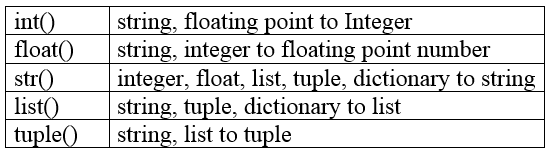# Python Type Conversion

Python has five standard Data Types. Sometimes it is necessary to convert values from one type to another. Python defines type conversion functions to directly convert one data type to another which is useful in day to day and competitive program development.## Python String to Integer

The method int() is the Python standard built-in function to convert a string into an integer value. You call it with a string containing a number as the argument, and it returns the number converted to an actual integer:

str =100 x = int(str) y = x+ 200 print(y)
output
300
example
x= "100" y="-50" z = int(x)+int(y) print(z)
output
50

### Python String to float

x= "10.5" y="4.5" z = float(x)+float(y) print(z)
output
15

### Python Floats to Integers

x = 10.5 y = 4.5 z = int(x) + int(y) print(z)
output
14

### Python Integers to Floats

x = 100 y = 200 z = float(x) + float(y) print(z)
output
300.0

### Python Float to string

x = 100.00 y = str(x) print(y)

## Converting to Tuples and Lists

1. A list is a mutable ordered sequence of elements that is contained within square brackets [ ].
2. A tuple is an immutable ordered sequence of elements contained within parentheses ( ).

You can use the methods list() and tuple() to convert the values passed to them into the list and tuple data type respectively.

### Python List to Tuple

lst = [1,2,3,4,5] print(lst) tpl = tuple(lst) print(tpl)
output
[1, 2, 3, 4, 5] (1, 2, 3, 4, 5)

### Python Tuple to List

tpl = (1,2,3,4,5) print(tpl) lst = list(tpl) print(lst)
output
(1, 2, 3, 4, 5) [1, 2, 3, 4, 5]

### ValueError

While converting from string to int you may get ValueError exception. This exception occurs if the string you want to convert does not represent any numbers.

### example

str = "halo" x = int(str) print(x)
output
Traceback (most recent call last): File "test.py", line 3, in < module > x = int(str) ValueError: invalid literal for int() with base 10: 'halo'

You can see, the above code raised a ValueError exception if there is any digit that is not belong to decimal number system.

try: str = "halo" x = int(str) except ValueError: print("Could not convert !!!")
output
Could not convert !!!

If you are ever unsure of the type of the particular object, you can use the type() function:

print(type('Hello World!')) print(type(365)) print(type(3.14))
output
< class 'str' > < class 'int' > < class 'float' >